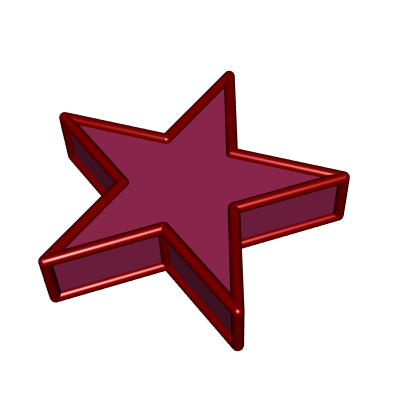# PolygonSoup

Make a consistent mesh from a polygon soup.

A polygon soup is a set of unorganized polygons. For example, define a tetrahedron as follows:

``````vertices <- rbind(
c(-1, -1, -1),
c( 1,  1, -1),
c( 1, -1,  1),
c(-1,  1,  1)
)
faces <- rbind(
c(1, 2, 3),
c(3, 4, 2),
c(4, 2, 1),
c(4, 3, 1)
)``````

Now plot it by painting the front of its faces in blue and the back of its faces in red; then both colors appear:

``````library(rgl)
tmesh <- tmesh3d(
vertices    = t(vertices),
indices     = t(faces),
homogeneous = FALSE
)
shade3d(tmesh, color = "blue", back = "cull")
shade3d(tmesh, color = "red", front = "cull")``````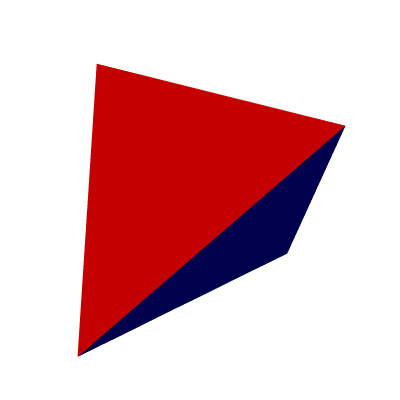To get a mesh with coherently oriented faces, use the `Mesh` function:

``````library(PolygonSoup)
mesh  <- Mesh(vertices, faces, normals = FALSE)
tmesh <- toRGL(mesh)
shade3d(tmesh, color = "blue", back = "cull")``````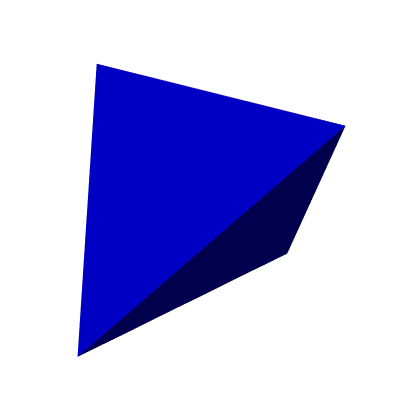The PolygonSoup package can deal with polygon soups whose polygons have any number of sides. For example, consider a pentagrammic prism: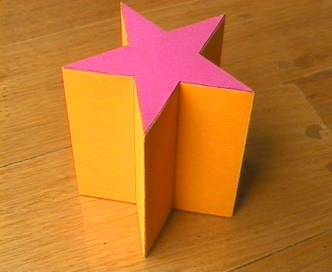The vertices and the faces of a pentagrammic prism are given in the package, in a list named `PentagrammicPrism`. Two faces, at the center of the two pentagramms, are pentagonal. Therefore one cannot directly plot the prism with rgl. The `Mesh` function allows to triangulate the faces:

``````mesh <- Mesh(
mesh = pentagrammicPrism,
triangulate = TRUE, normals = FALSE
)``````

Now we can plot the pentagrammic prism. We can also plot its edges with the help of the `plotEdges` function:

``````tmesh <- toRGL(mesh)
plotEdges(
mesh[["vertices"]], mesh[["edges"]], color = "darkred",
)``````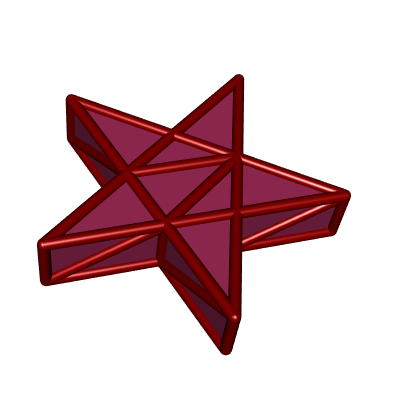The triangulation introduces additional edges, and you probably don’t want to see them. In `mesh[["edges0"]]`, one has the edges before the triangulation:

``````shade3d(tmesh, color = "maroon")
plotEdges(
mesh[["vertices"]], mesh[["edges0"]], color = "darkred",
)``````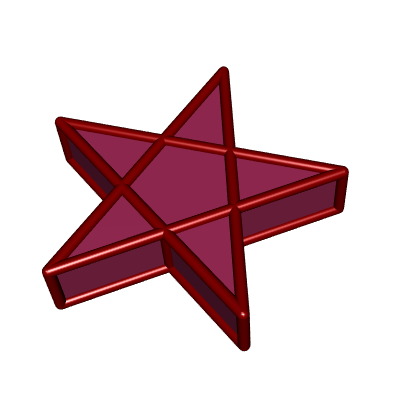One can also plot only the exterior edges, that is to say the edges which are not between two coplanar faces:

``````shade3d(tmesh, color = "maroon")
plotEdges(
mesh[["vertices"]], mesh[["exteriorEdges"]], color = "darkred",